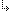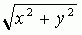- 4.45.2.33. -4. Standard Units4.45. Math - Extended Mathematics unit4.45.2. Math Unit Procedures and Functions4.45.2.33. Hypot function

## 4.45.2.33. Hypot function

Targets: MS-DOS, OS/2, Win32

Math Unit

Calculates the length of the hypotenuse.

Declaration:
`function Hypot(X, Y: Extended): Extended;`
Remarks:
The Hypot function returns the length of the hypotenuse of a right triangle. Specify the lengths of the sides adjacent to the right angle as X and Y. Hypot uses the formula:- 4.45.2.33. -